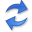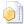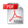Discovering Fully Oriented Causal Networks

Abstract. We study the problem of inferring causal graphs from observational data. We are particularly interested in discovering graphs where all edges are oriented, as opposed to the partially directed graph that the state-of-the-art discover. To this end we base our approach on the algorithmic Markov condition. Unlike the statistical Markov condition, it uniquely identifies the true causal network as the one that provides the simplest—as measured in Kolmogorov complexity—factorization of the joint distribution. Although Kolmogorov complexity is not computable, we can approximate it from above via the Minimum Description Length principle, which allows us to define a consistent and computable score based on non-parametric multivariate regression. To efficiently discover causal networks in practice, we introduce the Globe algorithm, which greedily adds, removes, and orients edges such that it minimizes the overall cost. Through an extensive set of experiments we show Globe performs very well in practice, beating the state-of-the-art by a margin.

## Implementationthe Python source code (August 2022) by Osman Ali Mian.

## Related PublicationsMian, OA, Marx, A & Vreeken, J Discovering Fully Oriented Causal Networks. In: Proceedings of the AAAI Conference on Artificial Intelligence (AAAI), AAAI, 2021. (21.3% acceptance)